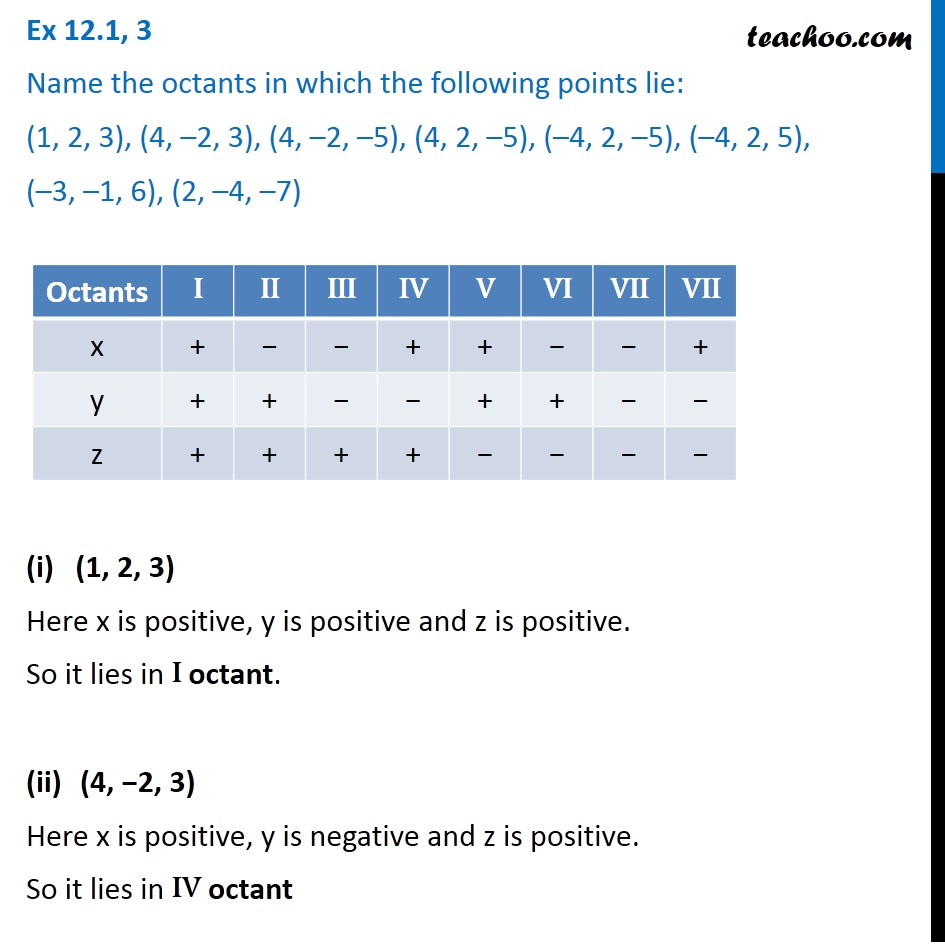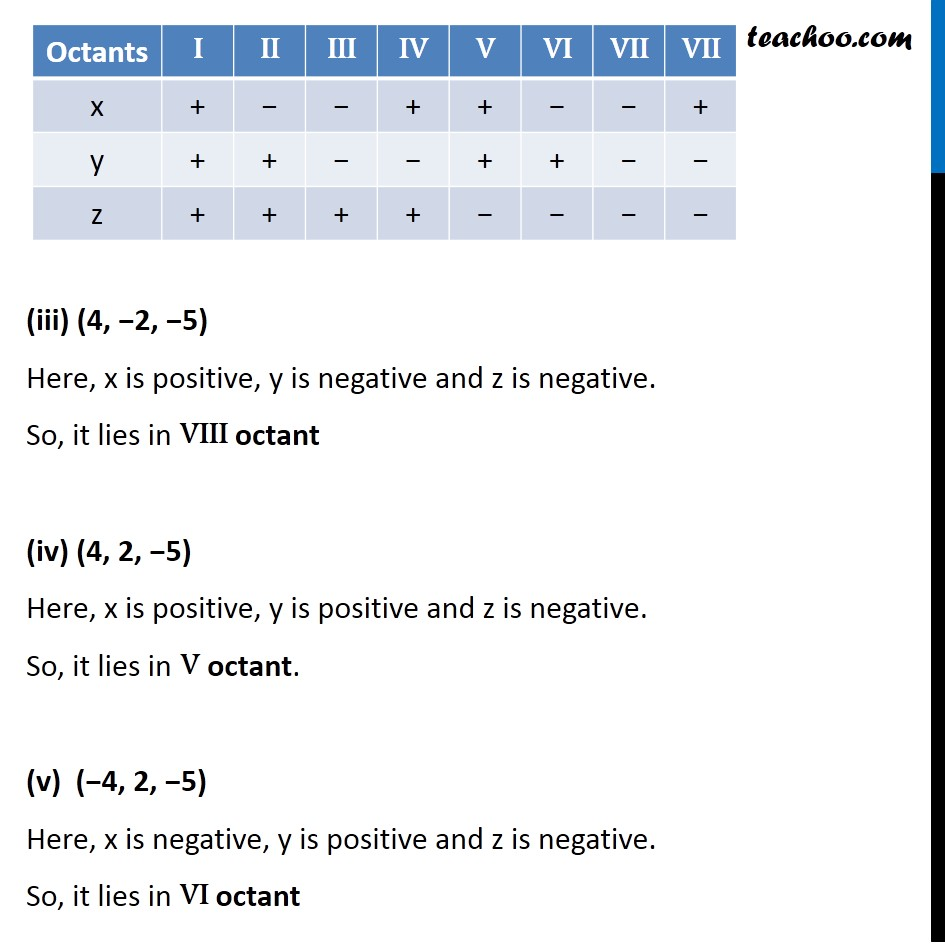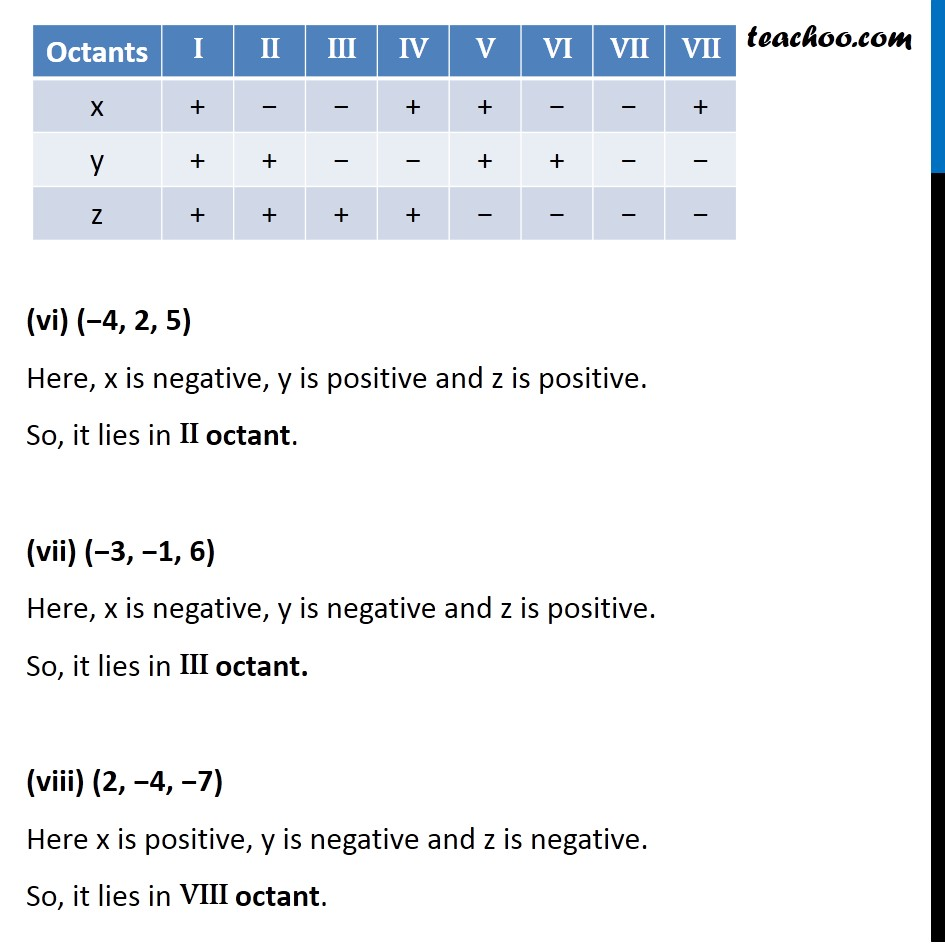Ex 12.1

Chapter 12 Class 11 - Intro to Three Dimensional Geometry (Term 2)
Serial order wise### Transcript

Ex 12.1, 3 Name the octants in which the following points lie: (1, 2, 3), (4, –2, 3), (4, –2, –5), (4, 2, –5), (–4, 2, –5), (–4, 2, 5), (–3, –1, 6), (2, –4, –7) (i) (1, 2, 3) Here x is positive, y is positive and z is positive. So it lies in I octant. (4, −2, 3) Here x is positive, y is negative and z is positive. So it lies in IV octant (iii) (4, −2, −5) Here, x is positive, y is negative and z is negative. So, it lies in VIII octant (iv) (4, 2, −5) Here, x is positive, y is positive and z is negative. So, it lies in V octant. (v) (−4, 2, −5) Here, x is negative, y is positive and z is negative. So, it lies in VI octant (vi) (−4, 2, 5) Here, x is negative, y is positive and z is positive. So, it lies in II octant. (vii) (−3, −1, 6) Here, x is negative, y is negative and z is positive. So, it lies in III octant. (2, −4, −7) Here x is positive, y is negative and z is negative. So, it lies in VIII octant.